Courses

# Crystals NAT Level – 2

## 10 Questions MCQ Test Topic wise Tests for IIT JAM Physics | Crystals NAT Level – 2

Description
This mock test of Crystals NAT Level – 2 for IIT JAM helps you for every IIT JAM entrance exam. This contains 10 Multiple Choice Questions for IIT JAM Crystals NAT Level – 2 (mcq) to study with solutions a complete question bank. The solved questions answers in this Crystals NAT Level – 2 quiz give you a good mix of easy questions and tough questions. IIT JAM students definitely take this Crystals NAT Level – 2 exercise for a better result in the exam. You can find other Crystals NAT Level – 2 extra questions, long questions & short questions for IIT JAM on EduRev as well by searching above.
*Answer can only contain numeric values
QUESTION: 1

### The probability that a state which is 0.2eV above the fermi energy in a metal atom at 700K is?

Solution:

E - Ef = 0.2 eV
kB = 8.62 x 10-5 eVK-1
T = 700K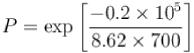P = 0.036

*Answer can only contain numeric values
QUESTION: 2

### Be has hexagonal closed packed structure with smaller lattice parameter equal to 2.29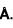. Concentration of electron in Be crystal is in terms of 1029 m-3 .

Solution:

Here a = 2.29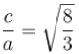for hexagonal close packing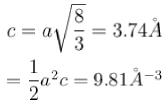There are two atoms in primitive cell and each atom carries 4 electrons.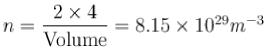*Answer can only contain numeric values
QUESTION: 3

### A copper wire of uniform cross-sectional area 10 x 10-6 m-2 carries a current of 1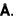Assuming that each copper atom contributes one electron to the electron gas, the drift velocity of free electron in units of µm/sec is :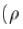of copper is 8.94 x 103 kg/m3 and its atomic mass is 1.05 x 10-25 kg).

Solution: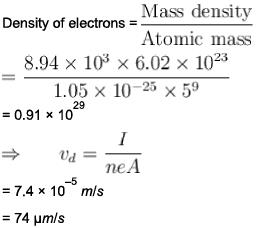*Answer can only contain numeric values
QUESTION: 4

Consider a crystal of fee structure. The density of the crystal is 8.96 glcm3 and atomic weight is 63.5. Then what is the lattice constant for the crystal (in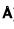)?

Solution:

Lattice constant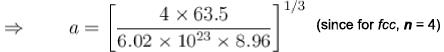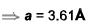*Answer can only contain numeric values
QUESTION: 5

The distance between the adjacent atomic planes in CaCO3 is 0.3 nm. The smallest angle of Bragg scattering for 0.03 nm X -ray is (in degrees)

Solution:

- For constructive interference, we must have

2dsinθ=nλ

sinθ=nλ/2d

=1×0.3×10−10 / 2×0.3×10-9

or

=0.05×180/π = 2.86degree

*Answer can only contain numeric values
QUESTION: 6

Sodium contains 2.54 x1022   free electrons per cm3 ( given = 6.63 x 10 -34 J-s, m= 9 .11 x 10-31 kg , kB = 1.38 x 10-23 JIK and 1eV = 1.6 x 10-19 J ) , then Fermi D 4 temperature is given in terms of 104 K as?

Solution:

We know that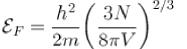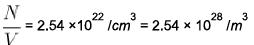⇒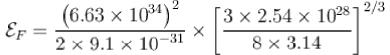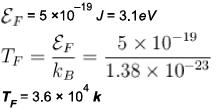*Answer can only contain numeric values
QUESTION: 7

In a powder diffraction pattern recorded from a face-centred cubic sample using X- rays. The first peak appears at 30°. The second peak will appear at what angle (degrees)

Solution: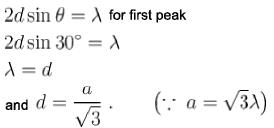For 2nd peak [2 0 0],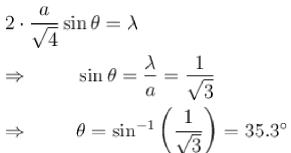*Answer can only contain numeric values
QUESTION: 8

Masses of ionic radii of Na+ and CI– in NaCI crystals are 22.99 amu, 0.98A and 35.45 amu, 1.82A respectively. What is the packing fraction of NaCI?

Solution:

Lattice parameter a =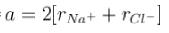a = 2 [0.98 + 1.82]
a = 5.6A
Each unit cell contains 4 atoms.
Packing fraction =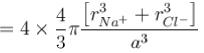Packing fraction =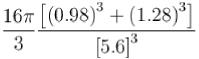Packing fraction = 0.66

*Answer can only contain numeric values
QUESTION: 9

Given that density of sodium is 0.971 g/cm3 and atomic weight is 23. If h = 6.63 * 10¯34 Js, mass of electron = 9.11 x 10-31 kg and Avogadro’s number is 6.02 x 1023 atoms/mol. Assuming each sodium atom contribute one electron to the electron gas. What is the electron density in units of 1028 Im3 ?

Solution:

Electron density of sodium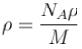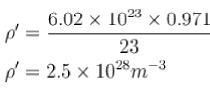*Answer can only contain numeric values
QUESTION: 10

Packing fraction of diamond cubic crystal structure is ____ % ?

Solution:

Number of atoms in diamond crystal
Packing fraction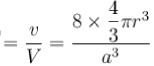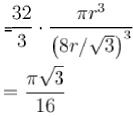= 0.34
= 34%